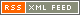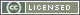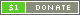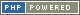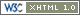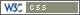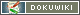Table of Contents

# Operators

An Operator can be thought of as an instruction that modifies one or more terms (values, or expressions - technically called "operands"), in order to produce another value. This operation is itself an expression.

For example:

`{ 4 + 5 }`

9

In the above example, the Operator `+` acts on the values `4` and `5` (which are literal constants). The `+` operator performs numeric addition, so the terms are added together and the expression is reduced to a single term, `9`, which is then displayed because there are no other instructions.

### Operator Types

PTPScript has three types of Operator, which between them work in two different ways. First there are infix Operators. These are "binary" operators, as they require an operand, or term, on both sides. Next there are "unary" operators, which only require a term on one side, so they are separated into prefix and postfix operators.

### Operator Precedence

When an expression is evaluated, the PTP engine looks through it from left to right. Each time an Operator is encountered, it is compared to the previous Operator (if there was one) to see which one is most important. The most important Operator therefore has the highest precedence. This is a key concept, because it affects the way that expressions are handled. Generally, things should act as commonly expected, but it is always good to know the order of precedence (see the Operator Predence Table). To change the way that an expression is handled, or to increase readability, use parentheses.

For example:

`{ 4 + 5 * 3 }`

19

The above example outputs `19`, because the numeric multiplication operator - `*` - has higher precedence than the numeric addition operator, so the expression is equivalent to `4 + (5 * 3)`. Use of parentheses will change this, i.e. `(4 + 5) * 3` gives `27`.

If two Operators have equal precedence, their associativity is checked, which then determines which will get evaluated first (for instance, the assignment operators have right-hand associativity, so everything to the right of the assignment operator is evaluated before the result is assigned to the Variable on the left of the operator).

For details of operator precedence and associativity, see the Operator Predence Tables.

ptpscript/operators.txt · Last modified: 2007/01/24 10:15